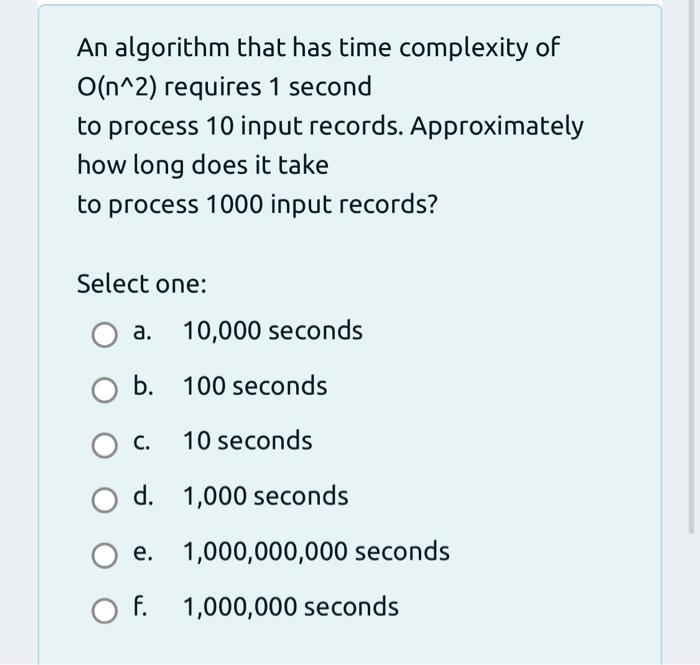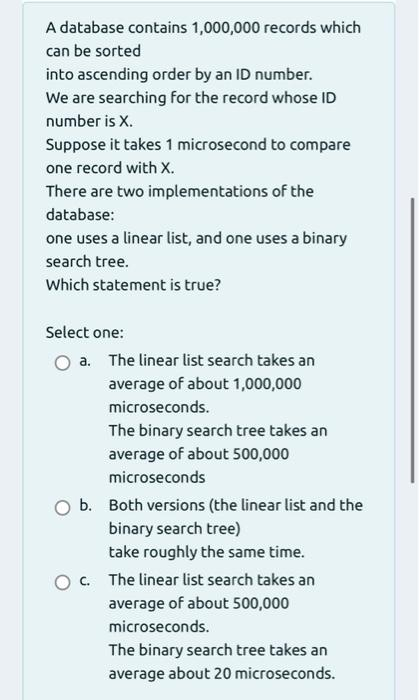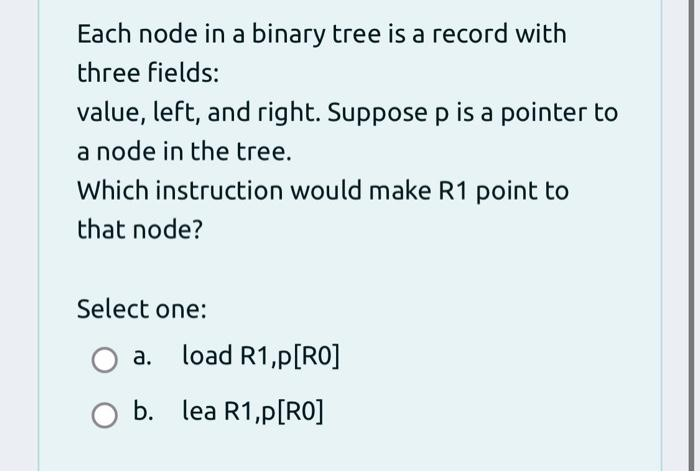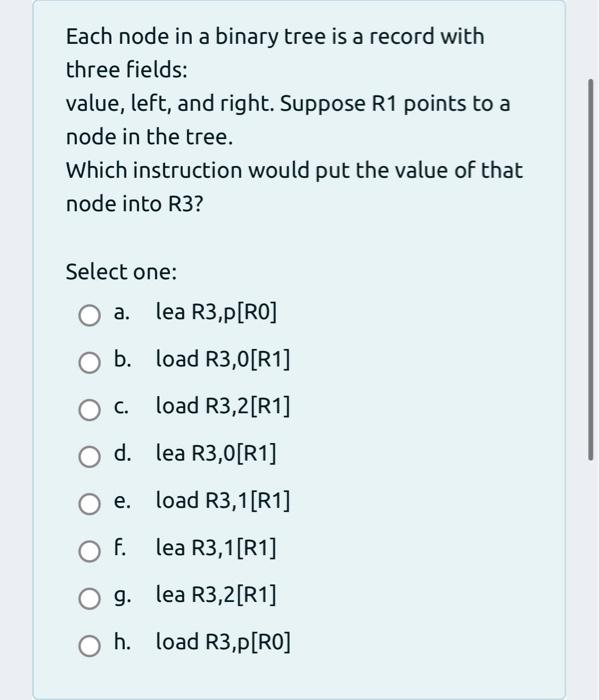# Question 3A)3B)3C)3D) An algorithm that has time complexity of O(n^2) requires 1 second to process 10 input records. Approximately how long does it take to process 1000 input records? Select one: a. 10,000 seconds b. 100 seconds O C. 10 seconds d. 1,000 seconds e. 1,000,000,000 seconds O F. f. 1,000,000 seconds A database contains 1,000,000 records which can be sorted into ascending order by an ID number. We are searching for the record whose ID number is X. Suppose it takes 1 microsecond to compare one record with X. There are two implementations of the database: one uses a linear list, and one uses a binary search tree. Which statement is true? Select one: a. The linear list search takes an average of about 1,000,000 microseconds. The binary search tree takes an average of about 500,000 microseconds b. Both versions (the linear list and the binary search tree) take roughly the same time. C. The linear list search takes an average of about 500,000 microseconds. The binary search tree takes an average about 20 microseconds. Each node in a binary tree is a record with three fields: value, left, and right. Suppose p is a pointer to a node in the tree. Which instruction would make R1 point to that node? Select one: : a. load R1,P[RO] b. lea R1,P[RO] Each node in a binary tree is a record with three fields: value, left, and right. Suppose R1 points to a node in the tree. Which instruction would put the value of that node into R3? Select one: a. lea R3,P[RO] b. load R3,0[R1] load R3,2[R1] C. d. lea R3,0[R1] load R3,1[R1] e. F. lea R3,1[R1] O g. lea R3,2[R1] O h. load R3,p[RO] ,TRY2NQ The Asker · Computer Science
3A)3B)3C)3D)Transcribed Image Text: An algorithm that has time complexity of O(n^2) requires 1 second to process 10 input records. Approximately how long does it take to process 1000 input records? Select one: a. 10,000 seconds b. 100 seconds O C. 10 seconds d. 1,000 seconds e. 1,000,000,000 seconds O F. f. 1,000,000 seconds A database contains 1,000,000 records which can be sorted into ascending order by an ID number. We are searching for the record whose ID number is X. Suppose it takes 1 microsecond to compare one record with X. There are two implementations of the database: one uses a linear list, and one uses a binary search tree. Which statement is true? Select one: a. The linear list search takes an average of about 1,000,000 microseconds. The binary search tree takes an average of about 500,000 microseconds b. Both versions (the linear list and the binary search tree) take roughly the same time. C. The linear list search takes an average of about 500,000 microseconds. The binary search tree takes an average about 20 microseconds. Each node in a binary tree is a record with three fields: value, left, and right. Suppose p is a pointer to a node in the tree. Which instruction would make R1 point to that node? Select one: : a. load R1,P[RO] b. lea R1,P[RO] Each node in a binary tree is a record with three fields: value, left, and right. Suppose R1 points to a node in the tree. Which instruction would put the value of that node into R3? Select one: a. lea R3,P[RO] b. load R3,0[R1] load R3,2[R1] C. d. lea R3,0[R1] load R3,1[R1] e. F. lea R3,1[R1] O g. lea R3,2[R1] O h. load R3,p[RO] ,
More
Transcribed Image Text: An algorithm that has time complexity of O(n^2) requires 1 second to process 10 input records. Approximately how long does it take to process 1000 input records? Select one: a. 10,000 seconds b. 100 seconds O C. 10 seconds d. 1,000 seconds e. 1,000,000,000 seconds O F. f. 1,000,000 seconds A database contains 1,000,000 records which can be sorted into ascending order by an ID number. We are searching for the record whose ID number is X. Suppose it takes 1 microsecond to compare one record with X. There are two implementations of the database: one uses a linear list, and one uses a binary search tree. Which statement is true? Select one: a. The linear list search takes an average of about 1,000,000 microseconds. The binary search tree takes an average of about 500,000 microseconds b. Both versions (the linear list and the binary search tree) take roughly the same time. C. The linear list search takes an average of about 500,000 microseconds. The binary search tree takes an average about 20 microseconds. Each node in a binary tree is a record with three fields: value, left, and right. Suppose p is a pointer to a node in the tree. Which instruction would make R1 point to that node? Select one: : a. load R1,P[RO] b. lea R1,P[RO] Each node in a binary tree is a record with three fields: value, left, and right. Suppose R1 points to a node in the tree. Which instruction would put the value of that node into R3? Select one: a. lea R3,P[RO] b. load R3,0[R1] load R3,2[R1] C. d. lea R3,0[R1] load R3,1[R1] e. F. lea R3,1[R1] O g. lea R3,2[R1] O h. load R3,p[RO] ,